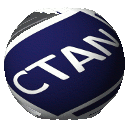# Annotate equations in LaTeX using TikZ

( Documentation | License: MIT | CTAN )

This package provides commands that make it easy to highlight terms in equations & add annotation labels (based on TikZ). Tested with pdflatex and lualatex, but should work with most other engines such as xelatex as well.

Inspired by Sibin Mohan's https://github.com/synercys/annotatedlatexequations/

Please report any bugs, issues, contribute changes & improvements on https://github.com/st–/annotate-equations/ :)

Package author: ST John, http://www.infinitecuriosity.org/

## A package with reusable commandsUsing annotate-equations.sty, an equation can easily be annotated as follows:

\begin{equation*}
\mathcal{O}\big(
(
\eqnmarkbox[NavyBlue]{p1}{p}
\eqnmarkbox[OliveGreen]{k1}{\kappa}^3  % note that we have the ^3 outside the \eqnmark/\tikzmarknode arguments
)
\eqnmarkbox[WildStrawberry]{T1}{T}
+
(
\eqnmarkbox[NavyBlue]{p2}{p}  % tikz nodes need distinct names!
\eqnmark[OliveGreen]{k2}{\kappa}
)
(
\eqnmarkbox[WildStrawberry]{T2}{T}^2
\tikzmarknode{Is}{|\mathcal{I}^*|}  % manual \tikzmarknode works, too
\eqnmarkbox[Plum]{Nb}{N_b}
\eqnmark[RoyalPurple]{M}{M}
)
\big)
\end{equation*}
\annotatetwo[yshift=1em]{above}{p1}{p2}{\# of nodes}
\annotatetwo[yshift=-1em,xshift=0.2ex]{below}{T1}{T2}{\# of graphs in $\hat{\mathcal{G}}_T$}
\annotatetwo[yshift=-2em]{below}{k1}{k2}{max.\ indegree in $\hat{\mathcal{G}}_T$}
\annotate[yshift=3em]{above,left}{Is}{size of set of allowed interventions}
\annotate[yshift=1em]{above}{Nb}{\# of samples per batch}
\annotate[yshift=-1em]{below}{M}{\# of samples for $\mathbb{E}_y$}


More details are in the user manual. Should work with both pdflatex and lualatex.

## How to do it from scratch: annotated examplesInspired by Sibin Mohan's https://github.com/synercys/annotatedlatexequations/, but with significantly simplified TikZ code, and with lots of comments to explain what is going on.

Two versions, one as Beamer slides with transitions, one as an article. For further examples, see https://github.com/synercys/annotatedlatexequations/.

### Giving all highlight boxes the same height

Simply add a \mathstrut within the colorbox:

-\newcommand{\highlight}{\colorbox{#1!17}{$#2$}}
+\newcommand{\highlight}{\colorbox{#1!17}{$\mathstrut #2$}}